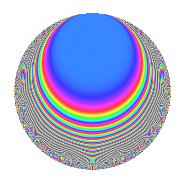# Properties

 Label 62.2.dLevel 62 Weight 2 Character orbit d Rep. character $$\chi_{62}(33,\cdot)$$ Character field $$\Q(\zeta_{5})$$ Dimension 16 Newform subspaces 2 Sturm bound 16 Trace bound 2

# Related objects

## Defining parameters

 Level: $$N$$ = $$62 = 2 \cdot 31$$ Weight: $$k$$ = $$2$$ Character orbit: $$[\chi]$$ = 62.d (of order $$5$$ and degree $$4$$) Character conductor: $$\operatorname{cond}(\chi)$$ = $$31$$ Character field: $$\Q(\zeta_{5})$$ Newform subspaces: $$2$$ Sturm bound: $$16$$ Trace bound: $$2$$ Distinguishing $$T_p$$: $$3$$

## Dimensions

The following table gives the dimensions of various subspaces of $$M_{2}(62, [\chi])$$.

Total New Old
Modular forms 40 16 24
Cusp forms 24 16 8
Eisenstein series 16 0 16

## Trace form

 $$16q - 6q^{3} - 4q^{4} - 4q^{5} + 8q^{6} - 2q^{7} - 12q^{9} + O(q^{10})$$ $$16q - 6q^{3} - 4q^{4} - 4q^{5} + 8q^{6} - 2q^{7} - 12q^{9} - 4q^{10} + 4q^{11} - 6q^{12} - 4q^{13} + 6q^{14} - 2q^{15} - 4q^{16} - 2q^{17} - 8q^{18} - 16q^{19} - 4q^{20} - 6q^{21} - 8q^{22} - 12q^{23} - 2q^{24} + 16q^{25} + 32q^{26} + 42q^{27} + 8q^{28} - 6q^{29} - 16q^{30} + 28q^{31} + 32q^{33} + 8q^{34} - 28q^{35} + 28q^{36} + 12q^{37} + 18q^{38} + 14q^{39} + 6q^{40} - 18q^{41} - 18q^{42} - 10q^{43} - 6q^{44} - 50q^{45} - 12q^{46} - 22q^{47} + 4q^{48} - 4q^{49} + 4q^{50} + 6q^{51} - 4q^{52} + 16q^{53} - 32q^{54} - 20q^{55} - 4q^{56} + 8q^{57} - 18q^{58} + 4q^{59} - 2q^{60} + 16q^{61} + 2q^{62} + 72q^{63} - 4q^{64} - 16q^{65} - 8q^{66} - 52q^{67} + 8q^{68} - 46q^{69} - 28q^{70} - 40q^{71} - 8q^{72} + 6q^{73} - 28q^{74} - 8q^{75} + 24q^{76} + 26q^{77} + 14q^{78} + 4q^{79} + 6q^{80} - 2q^{81} - 4q^{82} + 62q^{83} - 16q^{84} + 24q^{85} + 26q^{86} - 12q^{87} + 32q^{88} - 6q^{89} + 42q^{90} + 22q^{91} - 32q^{92} + 24q^{93} - 8q^{94} + 18q^{95} - 2q^{96} + 16q^{97} + 104q^{98} - 32q^{99} + O(q^{100})$$

## Decomposition of $$S_{2}^{\mathrm{new}}(62, [\chi])$$ into newform subspaces

Label Dim. $$A$$ Field CM Traces $q$-expansion
$$a_2$$ $$a_3$$ $$a_5$$ $$a_7$$
62.2.d.a $$8$$ $$0.495$$ 8.0.511890625.1 None $$-2$$ $$-4$$ $$-4$$ $$2$$ $$q-\beta _{2}q^{2}+(\beta _{1}-\beta _{2}+\beta _{3}+\beta _{4}-\beta _{5}+\cdots)q^{3}+\cdots$$
62.2.d.b $$8$$ $$0.495$$ 8.0.1903140625.1 None $$2$$ $$-2$$ $$0$$ $$-4$$ $$q-\beta _{6}q^{2}+\beta _{7}q^{3}+(-1+\beta _{2}+\beta _{3}+\cdots)q^{4}+\cdots$$

## Decomposition of $$S_{2}^{\mathrm{old}}(62, [\chi])$$ into lower level spaces

$$S_{2}^{\mathrm{old}}(62, [\chi]) \cong$$ $$S_{2}^{\mathrm{new}}(31, [\chi])$$$$^{\oplus 2}$$